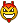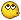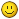8 years 10 months ago - 8 years 10 months ago #157 by mw0uzo
Replied by mw0uzo on topic Audio support for PyRadmon
my code has sinnedProbably best to get it working piece by piece rather than all at once.

1) Trim out all of the logic. Confirm you get a proper conversion from 16 bit signed integer to float by viewing the two waveforms. Perhaps dump the buffers out to a file to plot?
2) Add in the exponential function. Dump the buffer and confirm the envelope is correct
3) Then add in the pulse detection logic. Yes it stinks... but it worksYou're doing well. Sometimes I go back to my own code and think WTF?!! ... edit Sometimes? Often!
Last edit: 8 years 10 months ago by mw0uzo.

8 years 10 months ago #164
Yeah... Better idea indeed. xD
Well, I'll just fiddle around with it, then. ^_^

8 years 10 months ago #167 by mw0uzo
Replied by mw0uzo on topic Audio support for PyRadmon
If I get some time later on I'll take a look to see what might be wrong. Not familiar with python though, however your translations have illustrated some of the syntax. I find it quite weird coming from C, with the tabbed stuff etc, but its just another way of expressing { }. I wonder whether people would appreciate some graphical output in pyradmon? A window with the CPM graph and a window with the audio envelope? Perhaps python-graph is what to use. I know its extra for what is a cmd line tool, but it would be very useful for debugging.

8 years 10 months ago #169
Well, python is actually a direct descendant of C.
And indeed, as well as with python you will get indentation errors if you don't stick to the same indentation type (4 spaces or 1 tab, not mixed)
And the mathematical names are totally off compared to C#. :/
Here, a guide link for the maths within python. ^_^
https://docs.python.org/2/library/math.html

8 years 10 months ago #179
Found something that might aid in our journey to epicness.Got it from a Stack Overflow page.

With some tweaking and tidying and basically completely reworking the code to a usable state, we might pull this off.\
Snippet I found:
```#!/usr/bin/python

# open a microphone in pyAudio and listen for taps

import pyaudio
import struct
import math

INITIAL_TAP_THRESHOLD = 0.010
FORMAT = pyaudio.paInt16
SHORT_NORMALIZE = (1.0/32768.0)
CHANNELS = 2
RATE = 44100
INPUT_BLOCK_TIME = 0.05
INPUT_FRAMES_PER_BLOCK = int(RATE*INPUT_BLOCK_TIME)
# if we get this many noisy blocks in a row, increase the threshold
OVERSENSITIVE = 15.0/INPUT_BLOCK_TIME
# if we get this many quiet blocks in a row, decrease the threshold
UNDERSENSITIVE = 120.0/INPUT_BLOCK_TIME
# if the noise was longer than this many blocks, it's not a 'tap'
MAX_TAP_BLOCKS = 0.15/INPUT_BLOCK_TIME

def get_rms( block ):
# RMS amplitude is defined as the square root of the
# mean over time of the square of the amplitude.
# so we need to convert this string of bytes into
# a string of 16-bit samples...

# we will get one short out for each
# two chars in the string.
count = len(block)/2
format = "%dh"%(count)
shorts = struct.unpack( format, block )

# iterate over the block.
sum_squares = 0.0
for sample in shorts:
# sample is a signed short in +/- 32768.
# normalize it to 1.0
n = sample * SHORT_NORMALIZE
sum_squares += n*n

return math.sqrt( sum_squares / count )

class TapTester(object):
def __init__(self):
self.pa = pyaudio.PyAudio()
self.stream = self.open_mic_stream()
self.tap_threshold = INITIAL_TAP_THRESHOLD
self.noisycount = MAX_TAP_BLOCKS+1
self.quietcount = 0
self.errorcount = 0

def stop(self):
self.stream.close()

def find_input_device(self):
device_index = None
for i in range( self.pa.get_device_count() ):
devinfo = self.pa.get_device_info_by_index(i)
print( "Device %d: %s"%(i,devinfo["name"]) )

for keyword in ["mic","input"]:
if keyword in devinfo["name"].lower():
print( "Found an input: device %d - %s"%(i,devinfo["name"]) )
device_index = i
return device_index

if device_index == None:
print( "No preferred input found; using default input device." )

return device_index

def open_mic_stream( self ):
device_index = self.find_input_device()

stream = self.pa.open(   format = FORMAT,
channels = CHANNELS,
rate = RATE,
input = True,
input_device_index = device_index,
frames_per_buffer = INPUT_FRAMES_PER_BLOCK)

return stream

def tapDetected(self):
print "Tap!"

def listen(self):
try:
except IOError, e:
# dammit.
self.errorcount += 1
print( "(%d) Error recording: %s"%(self.errorcount,e) )
self.noisycount = 1
return

amplitude = get_rms( block )
if amplitude > self.tap_threshold:
# noisy block
self.quietcount = 0
self.noisycount += 1
if self.noisycount > OVERSENSITIVE:
# turn down the sensitivity
self.tap_threshold *= 1.1
else:
# quiet block.

if 1 <= self.noisycount <= MAX_TAP_BLOCKS:
self.tapDetected()
self.noisycount = 0
self.quietcount += 1
if self.quietcount > UNDERSENSITIVE:
# turn up the sensitivity
self.tap_threshold *= 0.9

if __name__ == "__main__":
tt = TapTester()

for i in range(1000):
tt.listen()```

Nice find